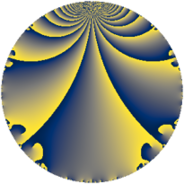# Properties

 Label 1815.2.a.dLevel $1815$ Weight $2$ Character orbit 1815.a Self dual yes Analytic conductor $14.493$ Analytic rank $1$ Dimension $1$ CM no Inner twists $1$

# Related objects

## Newspace parameters

 Level: $$N$$ $$=$$ $$1815 = 3 \cdot 5 \cdot 11^{2}$$ Weight: $$k$$ $$=$$ $$2$$ Character orbit: $$[\chi]$$ $$=$$ 1815.a (trivial)

## Newform invariants

 Self dual: yes Analytic conductor: $$14.4928479669$$ Analytic rank: $$1$$ Dimension: $$1$$ Coefficient field: $$\mathbb{Q}$$ Coefficient ring: $$\mathbb{Z}$$ Coefficient ring index: $$1$$ Twist minimal: no (minimal twist has level 15) Fricke sign: $$1$$ Sato-Tate group: $\mathrm{SU}(2)$

## $q$-expansion

 $$f(q)$$ $$=$$ $$q + q^{2} - q^{3} - q^{4} + q^{5} - q^{6} - 3q^{8} + q^{9} + O(q^{10})$$ $$q + q^{2} - q^{3} - q^{4} + q^{5} - q^{6} - 3q^{8} + q^{9} + q^{10} + q^{12} + 2q^{13} - q^{15} - q^{16} - 2q^{17} + q^{18} - 4q^{19} - q^{20} + 3q^{24} + q^{25} + 2q^{26} - q^{27} + 2q^{29} - q^{30} + 5q^{32} - 2q^{34} - q^{36} - 10q^{37} - 4q^{38} - 2q^{39} - 3q^{40} - 10q^{41} - 4q^{43} + q^{45} + 8q^{47} + q^{48} - 7q^{49} + q^{50} + 2q^{51} - 2q^{52} - 10q^{53} - q^{54} + 4q^{57} + 2q^{58} - 4q^{59} + q^{60} + 2q^{61} + 7q^{64} + 2q^{65} + 12q^{67} + 2q^{68} - 8q^{71} - 3q^{72} - 10q^{73} - 10q^{74} - q^{75} + 4q^{76} - 2q^{78} - q^{80} + q^{81} - 10q^{82} - 12q^{83} - 2q^{85} - 4q^{86} - 2q^{87} - 6q^{89} + q^{90} + 8q^{94} - 4q^{95} - 5q^{96} + 2q^{97} - 7q^{98} + O(q^{100})$$

## Embeddings

For each embedding $$\iota_m$$ of the coefficient field, the values $$\iota_m(a_n)$$ are shown below.

For more information on an embedded modular form you can click on its label.

Label $$\iota_m(\nu)$$ $$a_{2}$$ $$a_{3}$$ $$a_{4}$$ $$a_{5}$$ $$a_{6}$$ $$a_{7}$$ $$a_{8}$$ $$a_{9}$$ $$a_{10}$$
1.1
 0
1.00000 −1.00000 −1.00000 1.00000 −1.00000 0 −3.00000 1.00000 1.00000
 $$n$$: e.g. 2-40 or 990-1000 Significant digits: Format: Complex embeddings Normalized embeddings Satake parameters Satake angles

## Atkin-Lehner signs

$$p$$ Sign
$$3$$ $$1$$
$$5$$ $$-1$$
$$11$$ $$-1$$

## Inner twists

This newform does not admit any (nontrivial) inner twists.

## Twists

By twisting character orbit
Char Parity Ord Mult Type Twist Min Dim
1.a even 1 1 trivial 1815.2.a.d 1
3.b odd 2 1 5445.2.a.c 1
5.b even 2 1 9075.2.a.g 1
11.b odd 2 1 15.2.a.a 1
33.d even 2 1 45.2.a.a 1
44.c even 2 1 240.2.a.d 1
55.d odd 2 1 75.2.a.b 1
55.e even 4 2 75.2.b.b 2
77.b even 2 1 735.2.a.c 1
77.h odd 6 2 735.2.i.e 2
77.i even 6 2 735.2.i.d 2
88.b odd 2 1 960.2.a.l 1
88.g even 2 1 960.2.a.a 1
99.g even 6 2 405.2.e.c 2
99.h odd 6 2 405.2.e.f 2
132.d odd 2 1 720.2.a.c 1
143.d odd 2 1 2535.2.a.j 1
165.d even 2 1 225.2.a.b 1
165.l odd 4 2 225.2.b.b 2
176.i even 4 2 3840.2.k.r 2
176.l odd 4 2 3840.2.k.m 2
187.b odd 2 1 4335.2.a.c 1
209.d even 2 1 5415.2.a.j 1
220.g even 2 1 1200.2.a.e 1
220.i odd 4 2 1200.2.f.h 2
231.h odd 2 1 2205.2.a.i 1
253.b even 2 1 7935.2.a.d 1
264.m even 2 1 2880.2.a.y 1
264.p odd 2 1 2880.2.a.bc 1
385.h even 2 1 3675.2.a.j 1
429.e even 2 1 7605.2.a.g 1
440.c even 2 1 4800.2.a.bz 1
440.o odd 2 1 4800.2.a.t 1
440.t even 4 2 4800.2.f.bf 2
440.w odd 4 2 4800.2.f.c 2
660.g odd 2 1 3600.2.a.u 1
660.q even 4 2 3600.2.f.e 2

By twisted newform orbit
Twist Min Dim Char Parity Ord Mult Type
15.2.a.a 1 11.b odd 2 1
45.2.a.a 1 33.d even 2 1
75.2.a.b 1 55.d odd 2 1
75.2.b.b 2 55.e even 4 2
225.2.a.b 1 165.d even 2 1
225.2.b.b 2 165.l odd 4 2
240.2.a.d 1 44.c even 2 1
405.2.e.c 2 99.g even 6 2
405.2.e.f 2 99.h odd 6 2
720.2.a.c 1 132.d odd 2 1
735.2.a.c 1 77.b even 2 1
735.2.i.d 2 77.i even 6 2
735.2.i.e 2 77.h odd 6 2
960.2.a.a 1 88.g even 2 1
960.2.a.l 1 88.b odd 2 1
1200.2.a.e 1 220.g even 2 1
1200.2.f.h 2 220.i odd 4 2
1815.2.a.d 1 1.a even 1 1 trivial
2205.2.a.i 1 231.h odd 2 1
2535.2.a.j 1 143.d odd 2 1
2880.2.a.y 1 264.m even 2 1
2880.2.a.bc 1 264.p odd 2 1
3600.2.a.u 1 660.g odd 2 1
3600.2.f.e 2 660.q even 4 2
3675.2.a.j 1 385.h even 2 1
3840.2.k.m 2 176.l odd 4 2
3840.2.k.r 2 176.i even 4 2
4335.2.a.c 1 187.b odd 2 1
4800.2.a.t 1 440.o odd 2 1
4800.2.a.bz 1 440.c even 2 1
4800.2.f.c 2 440.w odd 4 2
4800.2.f.bf 2 440.t even 4 2
5415.2.a.j 1 209.d even 2 1
5445.2.a.c 1 3.b odd 2 1
7605.2.a.g 1 429.e even 2 1
7935.2.a.d 1 253.b even 2 1
9075.2.a.g 1 5.b even 2 1

## Hecke kernels

This newform subspace can be constructed as the intersection of the kernels of the following linear operators acting on $$S_{2}^{\mathrm{new}}(\Gamma_0(1815))$$:

 $$T_{2} - 1$$ $$T_{7}$$

## Hecke characteristic polynomials

$p$ $F_p(T)$
$2$ $$-1 + T$$
$3$ $$1 + T$$
$5$ $$-1 + T$$
$7$ $$T$$
$11$ $$T$$
$13$ $$-2 + T$$
$17$ $$2 + T$$
$19$ $$4 + T$$
$23$ $$T$$
$29$ $$-2 + T$$
$31$ $$T$$
$37$ $$10 + T$$
$41$ $$10 + T$$
$43$ $$4 + T$$
$47$ $$-8 + T$$
$53$ $$10 + T$$
$59$ $$4 + T$$
$61$ $$-2 + T$$
$67$ $$-12 + T$$
$71$ $$8 + T$$
$73$ $$10 + T$$
$79$ $$T$$
$83$ $$12 + T$$
$89$ $$6 + T$$
$97$ $$-2 + T$$